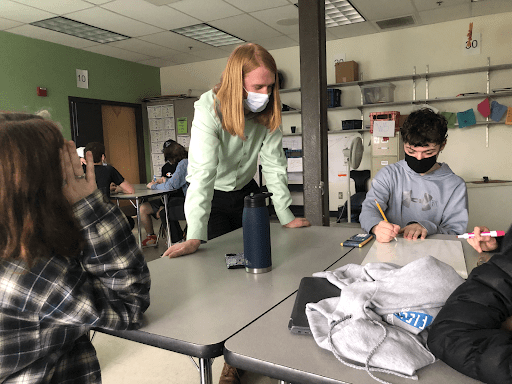# “It’s important they don’t just memorize the process” : Numeracy at U-32

This article was written by Wilder Gluck, a senior in the Journalism class at U-32.

At 1:36 on Tuesday, March 8th, 18 students took a math test. This test was a cumulation of math skills learned before Algebra Two: questions analyzing algebraic functions, basic statistics, complex fractions, and percentages.

Of these 18 students, 10 got 0/4 questions correct, 7 scored less than ¾. Only one student got a perfect 4/4.

Senior Sam Clark made some common mistakes on his test.

Given the task of simplifying ((2/7)+(5/6)-(7/3))/((4/7)-(1/6)+(5/3)) Clark was able to find a common denominator, giving him a result of (145/42)/(87/42)

But Clark added instead of subtracting the 7/3, and didn’t recognise that he could then cancel the denominators of each fraction to simplify further.

This is an example of a gap in numeracy, the general understanding of the functions of math. Sam needed to use proportional reasoning. He should have realized that the numerator should be a lower number than the denominator. He needed problem solving, since he tried to divide the top and bottom fractions before it was simplified enough to do so, and failed to find a common denominator to fully simplify his final answer.

Another common misunderstanding appeared in this problem:

“A news headline states ‘higher amounts of pets lead to lower stress levels.’ In the article it’s said that the correlation coefficient r=-.12. What is wrong with this claim? What would be a better headline?”

More than 75% of students got this question wrong. This shows a lack of understanding in statistics, and although students aren’t expected to understand complex statistics, it’s taught in Algebra One that the closer a correlation coefficient gets to -1 or 1, the stronger the relationship. With this knowledge, a student should be able to recognize the low value of -.12 suggests little or no correlation.

Despite the urge to blame these results on COVID, Hollis St. Peter, a middle school teacher at U-32, says he’s seen these holes for some time now.

U-32 has been tracking student understanding from middle school to 10th grade with diagnostic tests. Star 360 was used for 6 years, until it was replaced 3 years ago with I-Ready, a longer and more accurate test.

With this access to data showing students proficiency in specific skills, Hollis has been correcting misunderstandings through targeted study sessions with his 8th graders. “I’ve seen growth in all those kids,” Hollis said, “Almost 100% of them.”

Hollis said schools give kids calculators too early, and they should only be used once the concept is understood. “It’s super important that they don’t just memorize the process,” Hollis said, “but they understand what it is they’re doing.”

Without a fundamental understanding, incorrect calculator answers won’t be questioned. At this point, the calculator stops being a tool and becomes an obstacle.

In an attempt to fix these issues, the math department proposed a Math Functions class, which was decided against by the admin last year. “The issue isn’t that we need a class in between Algebra One and Algebra Two,” Drew, head of the math department, said, “It’s that we need to elevate what Algebra One is.”

Since that decision, Algebra One has changed from a 9th to a 10th-grade class. It focuses more on fractions and questioning skills, while working with tools like desmos, an online graphing calculator.

Other sites like “Get More Math” and “Mathematic” have been helping Algebra Two students with numeracy and proportional reasoning.

The Math Functions class also inspired a new class, Mathematical Modeling, which will be a semester-long class in the 22/23 U-32 curriculum. This class will help any student who’s passed Algebra Two build numeracy, problem-solving, statistics, and proportional reasoning skills, which will help them excel in AP math.